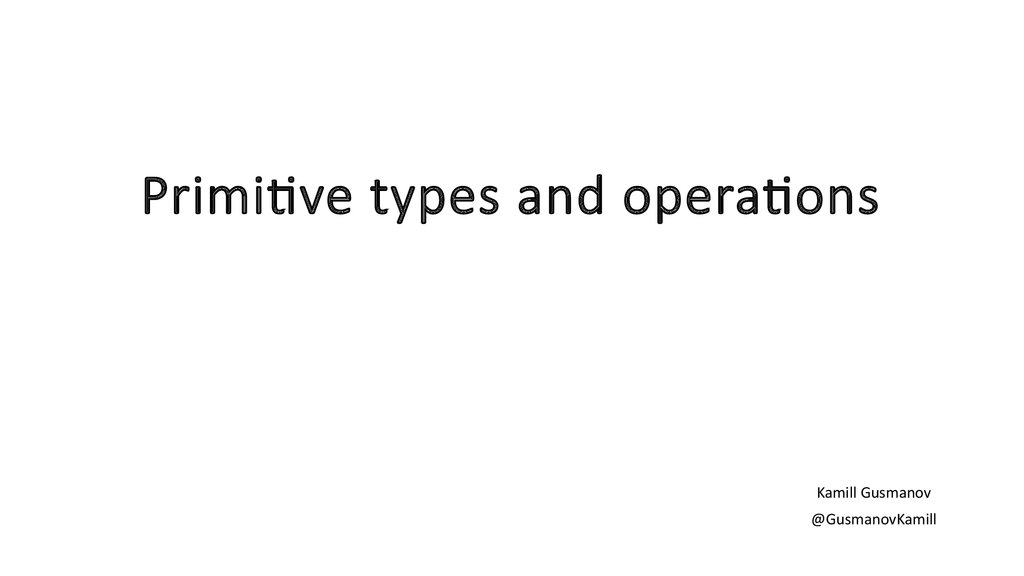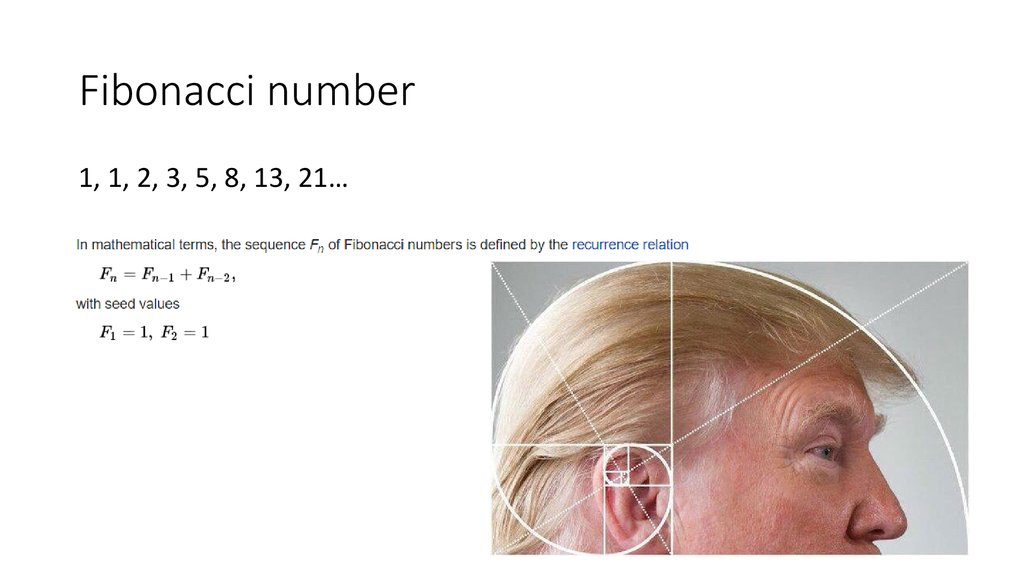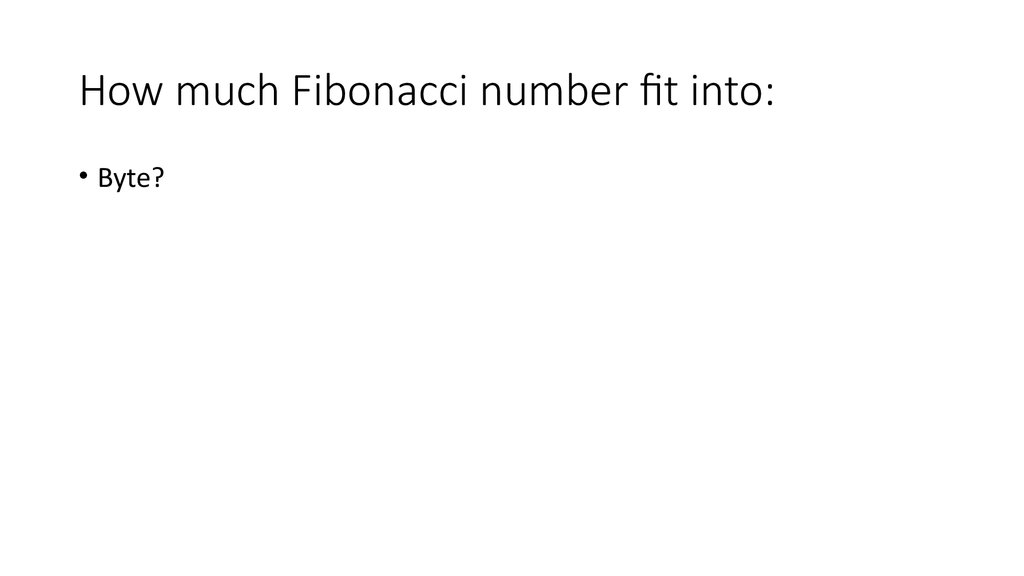# Primitive types and operations. Kamill Gusmanov @GusmanovKamill

Kamill Gusmanov
@GusmanovKamill

## 2. Fibonacci number

1, 1, 2, 3, 5, 8, 13, 21…

• Byte?

• Byte?
• Short?

• Byte?
• Short?
• Int?

• Byte?
• Short?
• Int?
• Long?

## 7. Random numbers

Unfortunately, this code is returning binary numbers as decimal integers, and you
cannot fix the library itself, but you can write a fix, that takes the result of the
function and converts it into regular integer. Use % and >> operations to complete

## 8. Arrays

Initialize array with Pascal triangle. Print it to the screen.

## 9. String

1. Multiply floating point numbers by 2 without floating point multiplication,
but using bitwise operations and Double.longBitsToDouble(long),
Double.doubleToRawLongBits(double)
long ld = Double.doubleToLongBits(d);
long sign = ld >> 63;
long exp = (ld >> 52) & 0x7FF;
long mantissa = ld & 0xFFFFFFFFFFFFFL;
System.out.println(sign);
System.out.println(exp - 1023);
System.out.println(1.0 + mantissa);